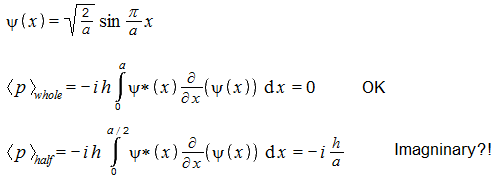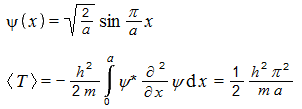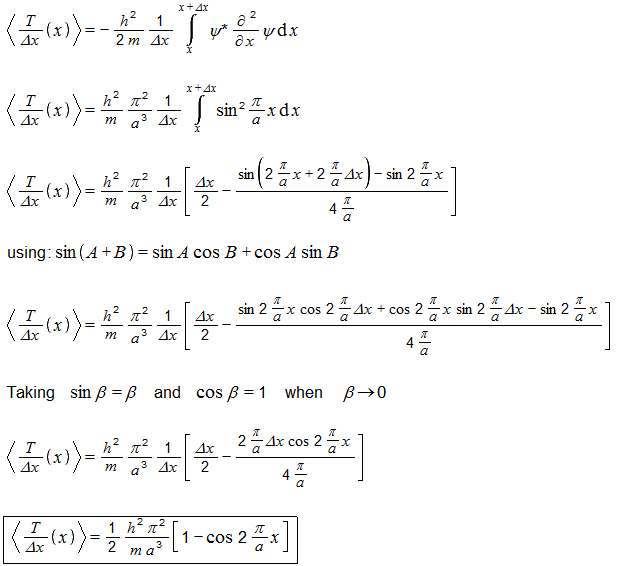## Recommended Posts

Hello... Say there is a particle in a 1D box. The particle is in certain state. I know its position-space wave function.

I can compute expectation value of its momentum by sandwiching the momentum operator between psi* and psi and integrating over the whole box width (or over whole infinity).

Question... If I don't integrate over the whole box, but only over a part of the box, can the result be interpreted as follows: if I simultaneously measure momentum and position of identically prepared setups, and if I only consider those cases where a particle was found within the part of the box for which I computed the integral, then the average momentum of those particles will equal to my integral?

##### Share on other sites
44 minutes ago, Danijel Gorupec said:

and if I only consider those cases where a particle was found within the part of the box for which I computed the integral,

##### Share on other sites
1 hour ago, studiot said:

So, from various sources this is an time-independent wave-function for a ground state particle in an infinite square (widht 'a'). The momentum calculation gives zero, as expected.But if I only integrate over half of the box width (the last line) then I get an imaginary result. Does this mean that this result has no interpretation (that it, it means nothing)?

[sorry, the 'h' should actually be h-bar, but I don't have this symbol]

Edited by Danijel Gorupec

##### Share on other sites

Hmm... but let's compute expectation value for kinetic energy... I am doing this for the same wave-function (ground state particle-in-a-box):

('h' is actually h-bar)The above is the correct result when the integral is computed over the whole box. But even if I integrate over only a part of the box, I still obtain real values - does it mean that such result might have some actual meaning?

Not being lazy, I tried to compute kinetic energy distribution (or density) over the box. Moderators can move this to the speculation forum.

I am using a sloppy notation as I know no better.This is the kinetic energy density function for a ground-state particle in a 1D box, how I calculated it.... Can this have any meaning? The function shape resembles the position probability density function for the particle.

I would be very glad if there is any function that can show kinetic energy density for wave-like particles. I like energy distributed in space (that is, local i like the local conservation of it). However, I never heard anything about it.

##### Share on other sites

What is the point of doing the integral over only part of the spatial extent?

## Create an account

Register a new account